Introduction : Integers

# Introduction : Integers - Notes | Study Additional Study Material for Class 7 - Class 7

 Table of contentsWhat are the Numbers?Basics of IntegersPositive NumbersHow do Operations work?Applications of Integers1 Crore+ students have signed up on EduRev. Have you?

What are the Numbers?
Any number is a symbol we use to denote a quantity are numbers.

Example: 2, 5, 99 etc.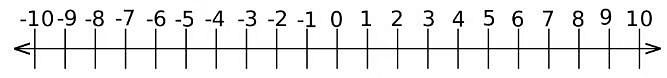Now, numbers are classified into various types:

Natural Numbers
Numbers used for counting in everyday life are called natural numbers eg. 2 pens, 6 chocolates etc. The addition of 2 natural numbers is a natural number, for example, 2 + 3 = 5. Also, the multiplication of 2 natural numbers is a natural number. For example, 2 × 3 = 6.

Whole Numbers

All the natural numbers along with zero (0) are called whole numbers. Therefore zero (0) is not a natural number as you cannot count zero. But zero is a whole number. Thus every natural number is a whole number. Zero is the only whole number that is not a natural number.

Addition of 2 whole number is a whole number, for example, 4 + 0 = 4. Also, the multiplication of 2 whole numbers is also a whole number, for example, 4 × 0 = 0.

Negative Numbers

Negative numbers are a concept i.e. they do not exist in reality. But for the purpose of calculation, we imagine a number system in which all natural numbers are preceded by a negative sign, for example, -1, -2, -3, -4….and so on. Their value is less than 0 and as the numbers increase the value of the unit decreases, for example -1 > -2 > -3 > -4 > -99 > -20000  and so on.

The addition of 2 negative numbers is also a negative number, for example (-2) +(-4) = (-6). Also the subtraction of a negative number may or may not be a negative number, for example (-2) -(-4) = -2 + 4 = 2.

Multiplication/ Division of 2 negative numbers is a positive number, for example -2 × -4 = 8;  -4 /-2 = 2. With these concepts clear, we now proceed to the main topic of our discussion.What is an Integer?

An integer is any number that is included in a set of combination of all the above numbers types. i.e. if we construct a set of all natural numbers, whole numbers, and negative numbers then such a set is called an Integer set, it can be represented as,

(……-3, -2, -1, 0, 1, 2, 3, ……)

So, every natural number is an integer, every whole number is an integer, and every negative number is an integer. The sum of 2 or more integers is an integer, for example, 2 + 4+ (-2) = 4. Also, the subtraction of 2 integers is also an integer, for example 2 + (-4) = -2. Similarly, the multiplication of 2 integers is also an integer, for example, 2 × -4 = -8.

The set of integers does not include fractions i.e p/q form or numbers which are in decimals eg: 2.4, 3.2 etc. The set of integers is an infinite set which means it has no ending on either side. That is because for every number on either the positive or negative side there is always one another number at an increment or decrement of 1 respectively. Hence the set of integers cannot be bound and is thus inexhaustible.

Example: Find 2 consecutive numbers whose sum is 129
Ans: Assume the numbers are ‘x’ and ‘x+1’
x + x + 1 = 129
so, 2x = 129 – 1
2x = 128
x = 64
if x = 64 then, x+1 = 65.
Hence, the numbers are 64 and 65.

Basics of Integers

Integer is a Latin word that means whole. An integer may comprise a set of whole numbers that include zero, positive number and negative number. Integers, however, do not include decimals, percents, and fractions. For understanding the basics of integers we need to represent it on a number line. The figure below shows numbers marked on a number line: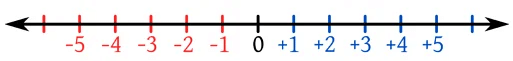The numbers on the right side of zero (0) are positive numbers while those on the left side are negative numbers. When we arrange these numbers in ascending order, the negative numbers are the lowest while the positive numbers are the highest. So in the number line above, amongst the numbers-5, 1 and 2 the number -5 is the lowest whereas 2 is the highest.

Positive Numbers

The numbers which have a plus (+) sign on them are called positive numbers. These numbers are present on the right of zero (0). Generally, a number without any sign is also considered a positive number. Every positive number is greater than its negative counterpart and zero. For example, 6 is greater than -6.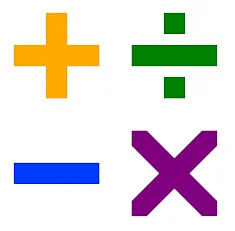Negative Numbers

A negative number is a contradiction of a positive number. It is written with a -ve sign. Negative numbers are present on the left of a zero on a number line. Every negative number is less than its positive counterparts.
Zero

A number that neither a positive nor a negative is a zero. It occupies the centre of the number line and is a neutral number.

How do Operations work?

When we use a number line to do mathematical operations like addition and subtraction we follow the underwritten points:

• When we add a positive integer, on a number line we move to the right. For example,  if we add 3 to-1 we move to the right on a number line.• When we add a negative integer on a number line we move to the left. For example, if we add -4 to +3 then moving left we get the answer -1.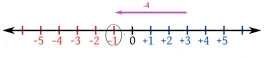• When we subtract a positive integer, we move to the left on that line. For example, when we subtract +4 from +2 we get -2.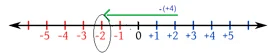• When we subtract a negative integer, on a number line we move to the right. For example, if we subtract -3 from -2 we get +1.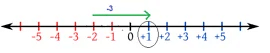• When we add one positive and one negative integer, then in such case we find the difference between the two integers. The sign, however, shall be the sign of the larger number. Her, we do not consider what the sign of larger number shall be for conducting the operation. This means that in any case, when there is a negative number, we have to subtract the lower from the higher.
• When we subtract two integers, we take them as the sum of one number with the additive inverse of that number. For example, if b has to be subtracted from a then we add the additive inverse of the integer b  to get the answer.  So when we subtract b from a, we write it as a + (-b)     [-b is the additive inverse of b].

Now suppose if b itself is a negative integer then, a-(-b) = a+b. This means that when we subtract the negative integer from a positive integer, we ultimately end up adding the two integers. A minus sign, when combined with a minus sign, becomes a positive. Let’s take ‘a’ as a negative integer now.

When we take ‘a’ as a negative integer and b also as a negative integer then, -a-(-b) = -a+b= b-a. So when both the integers are negative, we find the difference between the two to get the answer. The sign, however, shall be of the higher integer.

For further understanding, the basics of Integers, another concept to understand is the additive inverse. An additive inverse. An additive inverse of an integer is the number with the contrasting sign. For +4 the additive inverse is -4. For -5 additive inverse shall be +5.

Comparing Integers

When we compare integers, we need to keep in mind the sign before the integer. A negative integer, no matter what the number is lower than any positive number. For example when we compare the integers -5 and 3, -5 is lower and 3 is higher. A negative sign makes the number lower.
Applications of Integers

Integers are generally used to signify two contradicting situations. These are not just any numbers, they are numbers with signs. Positive numbers and negative numbers can have varying applications. The most real application of integers is measuring the temperature.

The positive and negative aspects of a temperature let us know, compare and measure the change in temperature. Apart from measuring temperature integers are used in the credits and debits calculation by the banks. Integers help in quantifying things in a better way.
Example If a and b are two integers with values +5 and -7 respectively, then what shall be the sum and difference between the two:
a. 12, 2
b. -2,12
c. -2,-12
d. 2,12
Sol: (b) Sum of a+b = +5 +(-7) = -2
a-b = +5-(-7) = +12

The document Introduction : Integers - Notes | Study Additional Study Material for Class 7 - Class 7 is a part of the Class 7 Course Additional Study Material for Class 7.
All you need of Class 7 at this link: Class 7

## Additional Study Material for Class 7

17 videos|26 docs|2 tests
 Use Code STAYHOME200 and get INR 200 additional OFF

## Additional Study Material for Class 7

17 videos|26 docs|2 tests

Track your progress, build streaks, highlight & save important lessons and more!

,

,

,

,

,

,

,

,

,

,

,

,

,

,

,

,

,

,

,

,

,

;